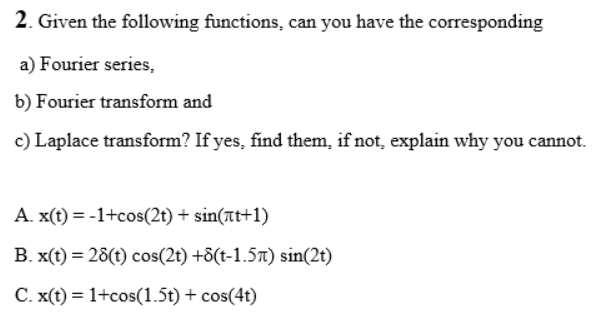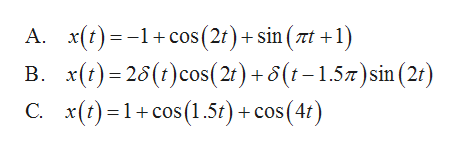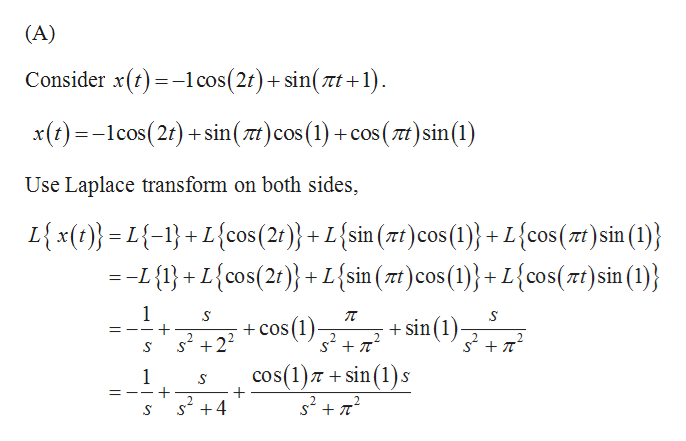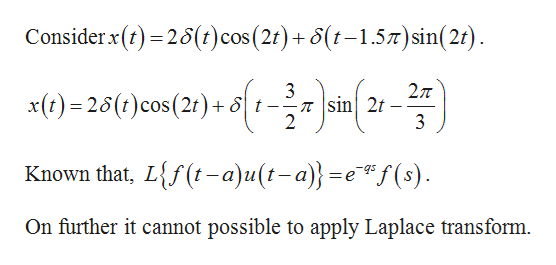# 2. Given the following functions, can you have the correspondinga) Fourier series,b) Fourier transform andc) Laplace transform? If yes, find them, if not, explain why you cannot.A. x(t) = -1+cos(2t) + sin(at+1)B. x(t) = 28(t) cos(2t) +8(t-1.57t) sin(2t)C. x(t) = 1+cos(1.5t) + cos(4t)%3D

Question
27 views

bhelp_outlineImage Transcriptionclose2. Given the following functions, can you have the corresponding a) Fourier series, b) Fourier transform and c) Laplace transform? If yes, find them, if not, explain why you cannot. A. x(t) = -1+cos(2t) + sin(at+1) B. x(t) = 28(t) cos(2t) +8(t-1.57t) sin(2t) C. x(t) = 1+cos(1.5t) + cos(4t) %3D fullscreen
check_circle

star
star
star
star
star
1 Rating
Step 1

From the given statement, the functions arehelp_outlineImage TranscriptioncloseА. х(t) 3—1+ cos(2t) + sin (zt + +1) В. (1)-26(г)сos(2) + 8(г-15л)sin (2:) С. х(г) -1+ сos (1.5t) + сos(4t) fullscreen
Step 2

Definition used:

A Fourier series is an expansion of a periodic function in terms of an infinite sum of sines and cosines.

The Laplace transforms a function of real variable t to a function of a complex variables s.

Calculation:

Note that, the range and period is not given in the functions.

So we can’t find Fourier series in this problem.

Calculation of Laplace transforms:help_outlineImage Transcriptionclose(A) Consider x(t) =-1cos(2t)+ sin( tt +1). x(t) =-1cos(2t) + sin( at)cos(1) +cos ( at) sin(1) Use Laplace transform on both sides, L{ x(t)} = L{-1}+ L{cos(2t)}+ L{sin (7t)cos (1)} + L{cos(rt)sin (1)} :-L{1}+ L{cos(2t)}+ L{sin ( rt)cos(1)}+L{cos(zt)sin (1)} + sin(1)- + cos (1)- 2. s´ +2? cos(1)7 + sin(1)s s + T s? +4 fullscreen
Step 3

Part (B)...help_outlineImage TranscriptioncloseConsider x(t) = 28(t)cos(2t)+8(t-1.57)sin(2t). 3 -T sin| 2t x(t) = 28(t)cos(2t) + 8 t- 3 Known that, L{f(t-a)u(t-a)}=e*f(s). On further it cannot possible to apply Laplace transform. fullscreen

### Want to see the full answer?

See Solution

#### Want to see this answer and more?

Solutions are written by subject experts who are available 24/7. Questions are typically answered within 1 hour.*

See Solution
*Response times may vary by subject and question.
Tagged in

### Math